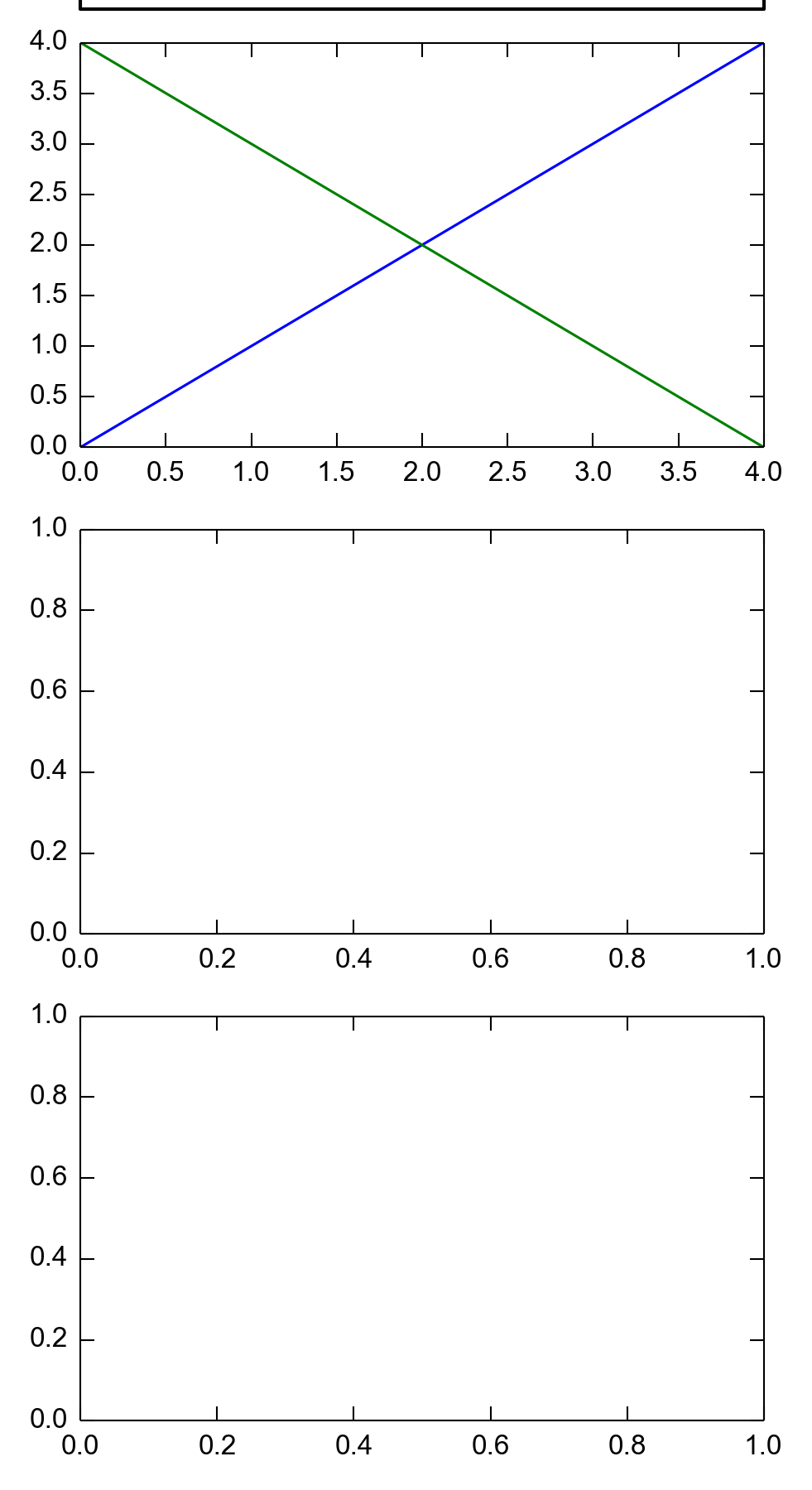# subplots_adjust in matplotlib does not work in IPython Notebook

Dear all,

I have the following code that did not work:···

################################
import matplotlib.pyplot as plt

# Make the plot
fig, axs = plt.subplots(3, 1, figsize=(3.27, 6))
axs.plot(range(5), range(5), label='label 1')
axs.plot(range(5), range(4, -1, -1), label='label 2')
axs.legend(bbox_to_anchor=(0, 1.1, 1., 0.1), mode='expand', ncol=2,

# Adjust subplots to make room

fig.savefig('test.png', format='png', dpi=300)
##################################

It can be seen that the fig.subplots_adjust did not work at all.
The picture from the notebook is normal, but the saved png file
(attached test.png) is cropped.

I am using WinPython 3.3.2.3 64 bit, with matplotlib version 1.3.0 and
CPython 3.3. This happened in IPython Notebook. The backend is the
in-line one.

Any help would really be appreciated. Thanks!

-Shawn

--
Yuxiang "Shawn" Wang
Gerling Research Lab
University of Virginia
yw5aj@...809...
+1 (434) 284-0836